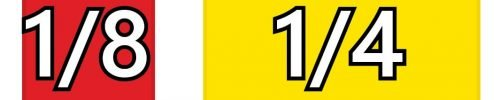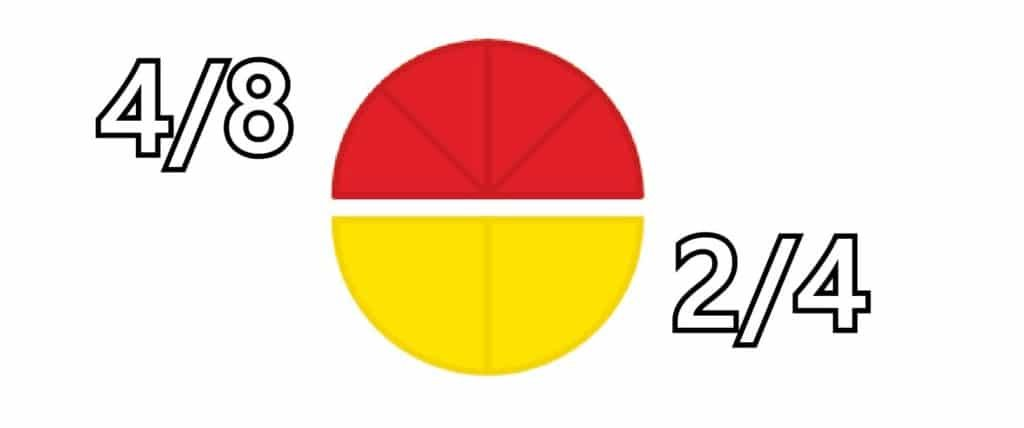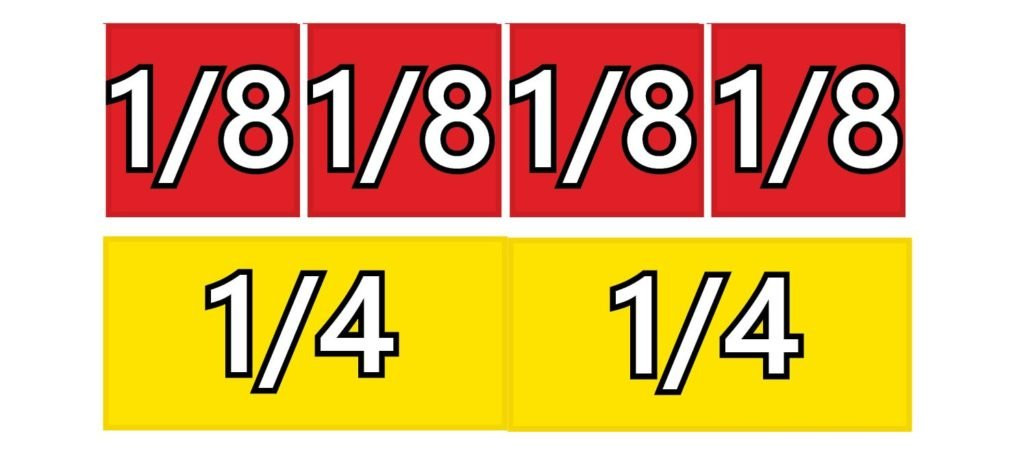# Fraction Manipulatives

#### • Number: Fractions

Fractions often cause difficulty for students and hence there is a plethora of manipulative materials designed to support the teaching and learning of fraction concepts. Early fraction teaching typically uses either length based or area based models to develop student understanding of fraction ideas such as the need to identify the unit and the need for parts of a unit to be the same size. It is for this reason that length based fraction manipulatives are recommended first. Rectangles and squares are simpler to partition or cut up either physically or mentally than circles.

### Mathematical Language

Decimal fraction, denominator, equivalent fraction, numerator.

Many students are confused by terms such as numerator and denominator. The denominator of the fraction refers to the name of the fraction. If it takes three equal size pieces to cover the whole piece then the name of the fraction is thirds.

If it takes six equal sized pieces to cover a whole then the fraction is named sixths. Each individual piece is one-sixth.

### Using Fraction Tiles And Circles

With fractions, the larger the denominator the smaller the piece. Consider the size of the 1/4 and the 1/8 piece. Both pieces are related to the same whole so we can compare them.Clearly the 1/8 is smaller than the 1/4 . Each of these fractions is a unit fraction, that is, there is a single piece – the numerator is one. Sometimes students are confused by the fact that the larger the denominator the smaller the piece. This is because they see the fraction as two separate whole numbers rather than a single number.

### Equivalent Fractions

Placing tiles end to end helps to establish the idea of equivalent fractions. For example, 2-fourths covers the same or an equivalent length as 4-eighths. This is the same length as 1-half. In fact 2/4 and 4/8 are other names for 1/2.Two-fourths, four-eighths are the same length as one-half. They are equivalent fractions.

### Typical Classroom Requirements

#### A class set:

A set of fraction bars per pair of students. A set of fraction circles per pair of students.